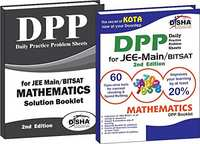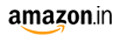Share

# Daily Practice Problem (DPP) Sheets for JEE Main/BITSAT Mathematics JEE MainM.R.P 375/-173.00(54%)
Product Description

The DPP Sheets are based on the concept of Prepare, Asses and improve.
Assessment is the most integral part of a student's preparation but still most of them avoid it. Assessment can only tell where you stand and how you can improve from that point. So it is very important that you take the right assessment, which is on the correct pattern, has the same level of difficulty as the actual exam and covers all the important concepts of the subject.

The book "Daily Practice Problem (DPP) Sheets for JEE Main/ AIPMT Mathematics" is precise, apt and tuned to all the requirements of a JEE Main aspirant.
No matter where you prepare from – a coaching or NCERT books or any other textbook/ Guide - Daily Practice Problem Sheets provides you the right assesment on each topic. Your performance provides you the right cues to improve your concepts so as to perform better in the final examination.

It is to be noted here that these are not tests but act as a checklist of student's learning and ability to apply concepts to different problems.
It is our strong belief that if an aspirant works hard on the cues provided through each of the DPP sheets he/ she can improve his/ her learning and finally the SCORE by at least 20%.

##### Book Features:
• Division of the complete JEE Main/ AIPMT syllabus into 60 Most important Topics. Each chapter has been broken into 2 or more topics
• Time Limit, Maximum Marks, Cut-off, Qualifying Score for each DPP Sheet
• Ultimate tool for Concept Checking & Speed Building
• Collection of 1750+ Standardised MCQ's that will of all variety of questions
• Unique & innovative way of Learning through Assessment
• 2 Separate Booklets - DPP Booklet & Solution Booklet
• The DPP Sheet covers all important Concepts of each Topic
• As per latest pattern & syllabus of JEE Main/ NEET exam
• Compliant to all boards of education
• Sets
• Relations & functions-1: Domain, Co-domain, Range, Classification of Function, Value of the Function
• Trigonometric functions-1: System of Measurement of angle, Trigonometrical ratios, Trigonometrical Ratio of Allied Angles, Sum & Difference formulae, Formulae for sum or difference into product conversion
• Trigonometric functions-2: Conditional trigonometrical identities, Series
• Trigonometric Equations
• Mathematical Induction
• Complex Number-1: Imaginary Number, Representation of a complex Number in Argand diagram, Algebraic operations with Complex Number, Conjugate of Complex Number, Modulus of a Complex Number
• Complex Number-2: Argument of a Complex Number, Amplitude of a Complex Number, Square root of a Complex Number, Equation solutions
• Quadratic Equations-1: Solution of Quadratic Equation, Nature of Roots, Sum and product of roots, Formation of an equation given roots, Condition for common roots
• Linear Quantities
• Permutations & Combinations-1: Fundamental principle of Multiplication, Addition, Combinations, Permutations, Permutations in which all things are not different, Permutations in which things may be repeated, Restricted Permutations, Permutation of numbers when given digits include zero
• Permutations & Combinations-2: Fundamental principle of Multiplication, Addition, Combinations, Permutations, in which all things are not different Permutations in which things may be repeated restricted permutations, Permutation of numbers when given digits include zero
• Binomial theorem-1: Binomial theorem for positive integral index, middle term, particluar term in the expansion, find a term from the end
• Binomial Theorem-2: Expansion for any index, Application of Binomial theorem
• Sequence and Series-1: Arithmetic progression and Geometric progression
• Sequence and Series-2: Arithmetico - Geometric progression, Harmonical progression
• Straight Line-1: Different Forms of The Equation of Straight Line, Reduction of General Form of Equations into Standard Forms, Position of a Point Relative to a Line, Angle Between two Straight Lines
• Straight Line-2: Equation of parallel and perpendicular lines, Equation of straight lines through a point and making angle with y = mx + c, Length of perpendicular, condition of Concurrency, Bisector of angle between two straight lines passing through the point of intersection of two lines, Homogeneous equation, General equation of second degree, Equation of lines joining the intersection points of line and curve to the origin
• Circle-1: Standard forms of equation of a circle, equation of a circle in some special cases, position of a point with respect to a circle, line and circle
• Circle- 2: Equation of tangent and normal, chord of contact, Director circle, position of two circles, equation of a chord, circle through the points of intersection, angle of intersection of two circles, common chord of two circles, pole and polar, radical axis and radical centre
• Parabola
• Ellipse
• Hyperbola
• Properties of Triangles
• Height and Distances
• 3D-Geometry-1: Distance Formula, Section Formula
• Limits
• Derivative-1: Derivative of sum, difference, product and quotient of functions, derivative of polynomial and trigonometric functions
• Statistics-1: Measures of Central Tendency
• Statistics-2: Measures of Dispersion
• Probability-1: Mathematical Definition of probability, Odds for an event, mutually exclusive events, theorem of probability
• Mathematical Reasoning
• Relations & Functions-2: Types of relations, Types of functions, Composition of functions, Inverse functions, Binary operations
• Inverse Trigonometric Functions
• Matrices - 1: Types of Matrices, Algebra of Matrices Matrices - 2: Special type matrices; Transpose, Adjoint and Inverse of matrices; Rank of Matrices and solution of the equations using matrix
• Determinants
• Continuity and Differentiability
• Exponential and Logarithmic Series
• Derivative-2: Differentiation of implicit function, parametric functions, logarithmic differentiation
• Derivative-3: Differentiation by substitution
• Derivative-4: Higher order derivative
• Applications of Derivatives - 1: Rate measure, tangent and normal)
• Applications of Derivatives - 2: Maxima and Minima
• Applications of Derivatives-3: Increasing and decreasing, Rolle's Theorem, Lagrange's Mean Value Theorem
• Indefinite Integral - 1: Fundamental Integration, Integration by Substitution
• Indefinite integral - 2: Integration by parts, Integral of the form Integral of the form
• Indefinite Integral-3: Integration of rational function by using partial fractions, Evaluation of various forms of integration
• Definite integral - 1: Fundamental Definite Integration, Definite Integration by Substitution
• Definite integral - 2: Properties of Definite Integration, Miscellaneous Integration
• Application of Integrals: Area bounded by region
• Differential Equations-1: Order and degree of differential equations, formation of differential equations, variable separable type differential equations
• Differential Equations - 2: Homogeneous differential equations
• Vector Algebra -1: Modulus of vector, Algebra of vectors, Scalar product of two vectors and its applications, Vector product of two vectors and its applications
• Vector Algebra-2: Scalar triple product and their applications, Vector triple product
• 3D Geometry 2: Direction cosines and direction ratios, Projection, Line
• 3D - Geometry 3: Plane, Line and Plane, Sphere
• Probability-2: Probability, odds in favour and odds against' addition, Theorem on conditional probability, Baye's theorem
• Probability - 3: Probability distribution, Bernouli Trials and Binomial distribution